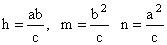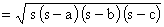# Geometry - Polygons

In geometry a polygon is traditionally a plane figure that is bounded by a closed path or circuit, composed of a finite sequence of straight line segments (i.e., by a closed polygonal chain). These segments are called its edges or sides, and the points where two edges meet are the polygon's vertices or corners. The interior of the polygon is sometimes called its body. A polygon is a 2-dimensional example of the more general polytope in any number of dimensions.

The word "polygon" derives from the Greek πολύς ("many") and γωνία (gōnia), meaning "knee" or "angle". Today a polygon is more usually understood in terms of sides. - Wikipedia

K = area
r= radius of inscribed circle
R = radius of circumscribed circle
p and q are diagonals
n= number of sides
θ = one of the vertex angles

 Right Triangle(Pythagorean)Equilateral TriangleRectangleParallelogramGeneral Quadrilateral(Bretschneider's Formula)Cyclic-Inscriptable QuadrilateralGeneral Trianglehc = length of altitude on side c,tc = length of bisector of angle C,mc = length of median to side c.(Law of Cosines)(Heron’s formula)RhombusTrapezoidRegular PolygonCyclic Quadrilateral(Brahmagupta’s formula)Source: CRC Standard Math Tables, 1987About RF CafeCopyright: 1996 - 2024Webmaster:    Kirt Blattenberger,    BSEE - KB3UON RF Cafe began life in 1996 as "RF Tools" in an AOL screen name web space totaling 2 MB. Its primary purpose was to provide me with ready access to commonly needed formulas and reference material while performing my work as an RF system and circuit design engineer. The Internet was still largely an unknown entity at the time and not much was available in the form of WYSIWYG ... All trademarks, copyrights, patents, and other rights of ownership to images and text used on the RF Cafe website are hereby acknowledged. My Hobby Website:  AirplanesAndRockets.com